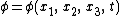# Laplace's irrotational motion

## Laplace's irrotational motion

Laplace's equation for irrotational motion of an inviscid, incompressible fluid is partial differential equation (1), where x1, x2, x3 are rectangular cartesian coordinates in an inertial reference frame, and Eq. (2)

(1)(2)gives the velocity potential. The fluid velocity components, u1, u2, u3 in the three respective rectangular coordinate directions are given by ui = ∂&phgr;/∂xi, i = 1, 2, 3. More generally, in any inertial coordinate system, the equation is div (grad &phgr;) = 0 and the velocity vector is v = grad &phgr;. Irrotational motion implies that the fluid particles translate without rotation (like the cars on a ferris wheel). See Fluid flow

Site: Follow: Share:
Open / Close